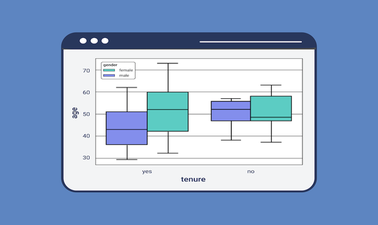# Introduction to Statistics for Data Science using Python

This Statistics for Data Science course is designed to introduce you to the basic principles of statistical methods and procedures used for data analysis.### There is one session available:

After a course session ends, it will be archived.
Starts Oct 15
Estimated 4 weeks
3–4 hours per week
Self-paced
Free

This Statistics for Data Science course is designed to introduce you to the basic principles of statistical methods and procedures used for data analysis. After completing this course you will have practical knowledge of crucial topics in statistics including - data gathering, summarizing data using descriptive statistics, displaying and visualizing data, examining relationships between variables, probability distributions, expected values, hypothesis testing, introduction to ANOVA (analysis of variance), regression and correlation analysis. You will take a hands-on approach to statistical analysis using Python and Jupyter Notebooks – the tools of choice for Data Scientists and Data Analysts.

At the end of the course, you will complete a project to apply various concepts in the course to a Data Science problem involving a real-life inspired scenario and demonstrate an understanding of the foundational statistical thinking and reasoning. The focus is on developing a clear understanding of the different approaches for different data types, developing an intuitive understanding, making appropriate assessments of the proposed methods, using Python to analyze our data, and interpreting the output accurately. This course is suitable for a variety of professionals and students intending to start their journey in data and statistics-driven roles such as Data Scientists, Data Analysts, Business Analysts, Statisticians, and Researchers. It does not require any computer science or statistics background. We strongly recommend taking the Python for Data Science course before starting this course to get familiar with the Python programming language, Jupyter notebooks, and libraries. An optional refresher on Python is also provided.

### At a glance

• Language: English
• Video Transcript: English

# What you'll learn

Skip What you'll learn

After completing this course, a learner will be able to:

• Calculate and apply measures of central tendency and measures of dispersion to grouped and ungrouped data.
• Summarize, present, and visualize data in a way that is clear, concise, and provides a practical insight for non-statisticians needing the results.
• Identify appropriate hypothesis tests to use for common data sets.
• Conduct hypothesis tests, correlation tests, and regression analysis.
• Demonstrate proficiency in statistical analysis using Python and Jupyter Notebooks.